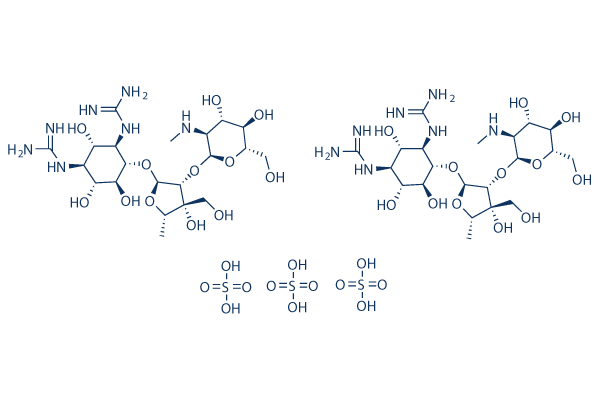# Dihydrostreptomycin sulfate

For research use only.

Catalog No.S4523CAS No. 5490-27-7

Dihydrostreptomycin sulfate is an aminoglycoside antibiotic, used to treat bacterial diseases in cattle, pigs and sheep. Dihydrostreptomycin is a derivative of streptomycin.

### Biological Activity

Description Dihydrostreptomycin sulfate is an aminoglycoside antibiotic, used to treat bacterial diseases in cattle, pigs and sheep. Dihydrostreptomycin is a derivative of streptomycin.
Targets
 MET channel 
In vitro

The primary mechanism of action of the antibiotic dihydrostreptomycin is binding to and modifying the function of the bacterial ribosome, thus leading to decreased and aberrant translation of proteins. It enters bacterial cells by binding to a specific site on MscL(mechanosensitive channel of large conductance) and modifying its conformation, thus allowing the passage of K+ and glutamate out of, and dihydrostreptomycin into, the cell.

In vivo Dihydrostreptomycin sulfate would cause cochlea damage in mouse model and cell proliferation in the spiral ligament may occur after the dihydrostreptomycin sulfate -induced damage. This process is probably related to the recovery of cochlear function.

### Solubility (25°C)

 In vitro Water 100 mg/mL (68.42 mM) DMSO Insoluble Ethanol Insoluble

* Please note that Selleck tests the solubility of all compounds in-house, and the actual solubility may differ slightly from published values. This is normal and is due to slight batch-to-batch variations.

Molecular Weight 1461.42 2C21H41N7O12.3H2SO4 5490-27-7 3 years-20°C powder 2 years-80°C in solvent N/A CC1C(C(C(O1)OC2C(C(C(C(C2O)O)N=C(N)N)O)N=C(N)N)OC3C(C(C(C(O3)CO)O)O)NC)(CO)O.CC1C(C(C(O1)OC2C(C(C(C(C2O)O)N=C(N)N)O)N=C(N)N)OC3C(C(C(C(O3)CO)O)O)NC)(CO)O.OS(=O)(=O)O.OS(=O)(=O)O.OS(=O)(=O)O

### In vivo Formulation Calculator (Clear solution)

 Step 1: Enter information below (Recommended: An additional animal making an allowance for loss during the experiment) Dosage mg/kgAverage weight of animals gDosing volume per animal ulNumber of animals Step 2: Enter the in vivo formulation (This is only the calculator, not formulation. Please contact us first if there is no in vivo formulation at the solubility Section.) % DMSO+ % PEG300 Corn oil + % Tween 80+ % ddH2O CalculateReset

### Bio Calculators

Molarity Calculator

## Molarity Calculator

Calculate the mass, volume or concentration required for a solution. The Selleck molarity calculator is based on the following equation:

### Mass (mg) = Concentration (mM) × Volume (mL) × Molecular Weight (g/mol)

• Mass
Concentration
Volume
Molecular Weight

*When preparing stock solutions, please always use the batch-specific molecular weight of the product found on the via label and SDS / COA (available on product pages).

Dilution Calculator

## Dilution Calculator

Calculate the dilution required to prepare a stock solution. The Selleck dilution calculator is based on the following equation:

### Concentration (start) x Volume (start) = Concentration (final) x Volume (final)

This equation is commonly abbreviated as: C1V1 = C2V2 ( Input Output )

• C1
V1
C2
V2

* When preparing stock solutions always use the batch-specific molecular weight of the product found on the vial label and SDS / COA (available online).

## The Serial Dilution Calculator Equation

• ### Computed Result

• C1=C0/X C2=C1/X C1: LOG(C1): C2: LOG(C2): C3: LOG(C3): C4: LOG(C4): C5: LOG(C5): C6: LOG(C6): C7: LOG(C7): C8: LOG(C8):
Molecular Weight Calculator

## Molecular Weight Calculator

Enter the chemical formula of a compound to calculate its molar mass and elemental composition:

### Total Molecular Weight: g/mol

Tip: Chemical formula is case sensitive. C10H16N2O2 c10h16n2o2

#### Instructions to calculate molar mass (molecular weight) of a chemical compound:

To calculate molar mass of a chemical compound, please enter its chemical formula and click 'Calculate'.

#### Definitions of molecular mass, molecular weight, molar mass and molar weight:

Molecular mass (molecular weight) is the mass of one molecule of a substance and is expressed in the unified atomic mass units (u). (1 u is equal to 1/12 the mass of one atom of carbon-12)
Molar mass (molar weight) is the mass of one mole of a substance and is expressed in g/mol.

#### Molarity Calculator

 Mass Concentration Volume Molecular Weight pg ng μg mg g kg = fM pM nM μM mM M × nL μL mL L ×

### Tech Support

Answers to questions you may have can be found in the inhibitor handling instructions. Topics include how to prepare stock solutions, how to store inhibitors, and issues that need special attention for cell-based assays and animal experiments.

Tel: +1-832-582-8158 Ext:3